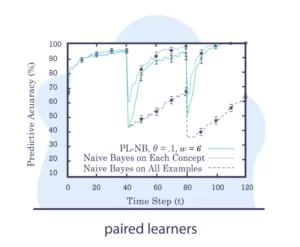To Glossary Lobby

# Paired Learners

Let’s say for a given problem we have a big stable model that uses a lot of data to train – let’s mark it as model A. We will also devise another model, a more lightweight model that trained on smaller and more recent data – it can have the same type. We’ll call it model B.

The idea: Find the time windows where model B outperforms model A. As model A is stable and encapsulates more data than model B, we would expect it to outperform it. However, if model B outperforms model A that might suggest that a concept drift has occurred.If interested, learn and read more about these concepts in our articles concept drift in machine learning 101 and 8 Concept Drift Detection Methods.A
B
C
D
E
F
M
N
P
R
S
T